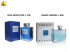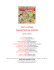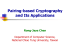# (1) Consider the differential equation 2xy0 â y = lny Find all solutions

## Transcription

(1) Consider the differential equation 2xy0 â y = lny Find all solutions
```(1) Consider the di↵erential equation
2xy 0
y = ln y 0 .
Find all solutions and the discriminant.
Solution: Let F (x, y, p) = 2xp y ln p, where p = y 0 . Let us consider the surface
M = {(x, y, p) : F (x, y, p) = 0} in the space of 1-jets. Solutions of the di↵erential equation
correspond to curves in M whose tangent vectors lie on the contact planes dy p dx = 0.
So, we need to find the integral curves of the following system:
F (x, y, p) = 0
dF = 0
=)
=)
dy
=)
p dx = 0
2p dx
2xp y
dy (2x
dy
Using dy = p dx, the second equation becomes p dx + (2x
p2 dx + (2xp
1
) dx
p
ln p = 0
=0
1
) dp
p
p dx = 0.
= 0. Multiplying by p gives
1) dx = 0.
That is,
d(p2 x
2
Thus, p x
p⌘
p) = 0.
1
C,
4
where C is a constant. Solving for p gives
p
1 ± 1 + Cx
p=
.
2x
Using the first equation, y = 2xp ln p, we find
p
✓
◆
p
1 ± 1 + Cx
y = 1 ± 1 + Cx ln
.
2x
The criminant is the set of points in the surface M = {(x, y, p) : F (x, y, p) = 0} such
that @F
= 0. That is, the set of points in the 1-jet space such that
@p
2xp
2x
y = ln p
1
= 0.
p
1
The second equation gives x = 2p
. Plugging this into the first equation gives y = 1 ln p.
Thus, the criminant can be described by the parametric curve in the 1-jet space given by
x(t) = 2t1
y(t) = 1
z(t) = t.
ln t
The discriminant is the projection of the criminant onto the xy-plane via (x, y, p) 7!
(x, y). This results in the parametric curve in the xy-plane given by
x(t) = 2t1
y(t) = 1
ln t.
One can also describe this curve as the graph of y = 1 + ln(2x). ⌃
(2) Find a curve on the plane whose tangent lines form with the coordinate axis triangles of
area 2a2 .
Remark: The following two solutions are ultimately equivalent.
Solution 1: The area of the triangle formed by the coordinate axes and the line Y = mX + b
2
is A = 12 bm . The tangent line to a curve Y = f (X) at a point (x, y) is given by Y =
f 0 (x)X + (f (x) xf 0 (x)). Thus, we require that
1 (f (x) xf 0 (x))2
= 2a2 ,
2
f 0 (x)
or equivalently,
xf 0 (x))2 = (2a)2 |f 0 (x)|.
(f (x)
Letting y = f (x) and p = f 0 (x), we have (y
px)2 = (2a)2 |p|, and hence
p
px = ±2a |p|.
y
p
Let F (x, y, p) := y px ± 2a |p|. Solutions to the di↵erential equation correspond to
curves in M = {(x, y, p) : F (x, y, p) = 0} whose tangent vectors lie on the contact planes
dy p dx = 0. Thus, we have
0 = dF =
p dx + dy + ( x ± pa sgn(p)) dx.
=( x±
pa
|p|
|p|
sgn(p)) dx.
This implies that x = ± pa sgn(p), so that x2 =
|p|
so that
f (x) = ±
a2
,
|p|
a2
.
x
so |p| =
a2
.
x2
2
In other words, f 0 (x) = ± xa2 ,
⌃
Solution 2: We will use the fact that every curve y = f (x) is the envelope of its family of
tangent lines.
Note first (cf Arnold: page 20) that the envelope of a family of lines {y = px g(p)}p2R
is the curve y = f (x), p
where f is the Legendre transform of g. Second, note that the family
of lines {y = px ± 2a |p|}p2R form triangles of area 2a2 with
p the coordinate axes. Thus,
the required curve is the Legendre transform of g(p) = ±2a |p|. p
@
To calculate the Legendre transform of g, we set @p
(px ± 2a |p|) = 0, which yields
2
2
a
a
a
x = ⌥ p , so |p| = x2 , so p = ± x2 . Thus,
|p|
f (x) = px ± 2a
p
|p| = ±
a2 2a2
a2
⌥
= ⌥ .
x
x
x
⌃
(3) Prove that the rank of any skew-symmetric bilinear form is even.
Solution 1 (Sketch): Show that the eigenvalues of any skew-symmetric bilinear form are
either zero or pure imaginary, and that the eigenvalues come in complex-conjugate pairs.
Thus, the number of non-zero eigenvalues – i.e., the rank of the bilinear form – is even. ⌃
Solution 2: Let ! be a skew-symmetric bilinear form on a finite-dimensional vector space V .
The result will follow from the following structure theorem.
Theorem: There exists a basis for V , denoted {u1 , . . . , uk , e1 , . . . , em , f1 , . . . , fm }, for which
!(ui , v) = 0, 8v 2 V
!(ei , ej ) = 0
!(fi , fj ) = 0
!(ei , fj ) = ij .
Such a basis is called a symplectic basis.
Proof of Theorem: Let U := {u 2 V : !(u, v) = 0, 8v 2 V } denote the nullspace of !. Let
{u1 , . . . , uk } be a basis of U . Write
V =U
W.
We will (inductively) decompose W into a direct sum of 2-dimensional subspaces. If W = 0,
we’re done, so assume W 6= 0.
Let e1 2 W , e1 6= 0. Since e1 2 W , there exists f1 2 W with !(e1 , f1 ) 6= 0. By rescaling,
we may assume that !(e1 , f1 ) = 1.
Let W1 := span{e1 , f1 }. Let W1! := {w 2 W : !(w, v) = 0, 8v 2 W1 } denote the
symplectic complement to W1 in W , so that
W = W1
W1! .
Let e2 2 W1! , e2 6= 0. Then there exists f2 2 W1! with !(e2 , f2 ) 6= 0. By rescaling, we
may assume that !(e2 , f2 ) = 1.
Repeat this process. This process eventually terminates because dim V < 1. Thus, we
have a direct sum decomposition
V =U
span{e1 , f1 }
···
span{em , fm }.
⌃
With respect to a symplectic basis for !, the skew-symmetric bilinear form ! can be
written as
0
1
0k
0
Idm A v.
!(u, v) = uT @
Idm 0
That is, with respect to a symplectic basis, the form ! is represented by the matrix
0
1
0k
@
0
Idm A,
Idm 0
which has rank 2m. In other words, rank(!) = 2m is even. ⌃
(4) Let us view Cn as R2n with the operation of multiplication by i. Prove that
SO(2n) \ Sp(2n; R) = GL(n; C) \ SO(2n) = GL(n; C) \ Sp(2n; R) = U(n).
Notation: Let g denote the standard inner product, let ! denote the standard symplectic
form, and let h denote the standard Hermitian form.
We let z, w 2 Cn denote
X
z = (x1 , . . . , xn , y 1 , . . . , y n ) =
xk + iy k
X
w = (u1 , . . . , un , v 1 , . . . , v n ) =
uk + iv k .
Solution 1 (Sketch): Recall from basic linear algebra that
SO(2n) = {A 2 GL(2n; R) : AT A = Id and detR (A) = 1}
U(n) = {A 2 GL(n; C) : A⇤ A = Id}.
Let J denote the matrix
◆
0
Idn
J=
.
Idn
0
We observe that the matrix J corresponds to multiplication by i. That is,
0 11
0 11 0
1
x
x
y1
B .. C
B .. C B .. C
.C B . C
B.C ✓
◆B
B nC
B nC B nC
X
0
Idn Bx C B y C
Bx C
Jz = J B 1 C =
xk + iy k = iz.
B 1C = B
C=i
Idn
0
By C
B y C B x1 C
B.C
B.C B . C
@ .. A
@ .. A @ .. A
yn
yn
xn
We observe also that J T =
is,
✓
J is the matrix representation of ! in the standard basis. That
!(z, w) = z T J T w.
From these facts, it follows that
GL(n; C) = {A 2 GL(2n; R) : AJ = JA}
Sp(2n; R) = {A 2 GL(2n; R) : AT JA = J}.
Using these four descriptions of the matrix groups SO(2n), U(n), GL(n; C), Sp(2n; R),
the first three desired intersections are now fairly immediate. For example:
If A 2 GL(n; C) \ O(2n), then JA = AJ and AT A = Id, so AT JA = AT AJ = J, so
A 2 Sp(2n; R). Thus, O(2n) \ GL(2n; C) ⇢ Sp(n; R). Analogous arguments hold for the
other two.
If A 2 U(n), then A 2 GL(n; C) and g(Az, Az) = h(Az, Az) = h(z, z) = g(z, z), so
g 2 O(2n), so A 2 Sp(2n; R) by the preceding paragraph, and so A lies in any of these
pairwise intersections.
Conversely, if A 2 GL(n; C) \ SO(2n), then h(Az, Az) = g(Az, Az) = g(z, z) = h(z, z),
hence (by polarization) h(Az, Aw) = h(z, w), so A 2 U(n). ⌃
(4) Let us view Cn as R2n with the operation of multiplication by i. Prove that
SO(2n) \ Sp(2n; R) = GL(n; C) \ SO(2n) = GL(n; C) \ Sp(2n; R) = U(n).
Notation: Let g denote the standard inner product, let ! denote the standard symplectic
form, and let h denote the standard Hermitian form.
We let z, w 2 Cn denote
X
z = (x1 , . . . , xn , y 1 , . . . , y n ) =
xk + iy k
X
w = (u1 , . . . , un , v 1 , . . . , v n ) =
uk + iv k .
Solution 2 (Sketch): Let us observe first that
X
X
h(z, w) =
zk wk =
(xk + iyk )(uk ivk )
X
=
(xk uk + yk vk ) + i(yk vk
= g(z, w)
x k uk )
i!(z, w).
(1)
From this, we observe secondly that
!(z, w) + ig(z, w) = ih(z, w) = h(iz, w) = g(iz, w)
i!(iz, w),
and so by equating real parts, we obtain
g(iz, w) = !(z, w)
(2)
Using equations (1) and (2), the result is now straightforward to check. ⌃
If A 2 SO(2n) \ Sp(2n; R), then h(Az, Aw) = g(Az, Aw) i!(Az, Aw) = g(z, w)
i!(z, w) = h(z, w), so A 2 U(n). Thus, SO(2n) \ Sp(2n; R) ⇢ U(n).
If A 2 U(n), then g(Az, Aw) i!(Az, Aw) = h(Az, Aw) = h(z, w) = g(z, w) i!(z, w),
so equating real and imaginary parts shows that A 2 SO(2n) \ Sp(2n; R). Thus, U (n) ⇢
SO(2n) \ Sp(2n; R).
This shows that U(n) = SO(2n) \ Sp(2n; R).
If A 2 GL(n; C) \ SO(2n), then !(Az, Aw) = g(iAz, Aw) = g(A(iz), Aw) = g(iz, w) =
!(z, w), so ! 2 Sp(2n; R). Thus, GL(n; C) \ SO(2n) ⇢ U(n).
If A 2 GL(n; C) \ Sp(2n; R), then g(Az, Aw) = !(iAz, Aw) = !(A(iz), Aw) =
!(iz, w) = g(z, w), so A 2 SO(2n). Thus, GL(n; C) \ Sp(2n; R) ⇢ U(n).
Finally, if A 2 U(n) = SO(2n) \ Sp(2n; R), then
g(iAz, Aw) = !(Az, Aw) = !(z, w) = g(iz, w) = g(A(iz), Aw),
so g(iAz Aiz, Aw) = 0. Since g is non-degenerate, this forces A(iz) = i(Az), so A 2
GL(n; C). ⌃
(5) Prove that the plane fields given by equations dz y dx = 0 and dz 12 (x dy y dx) = 0 are
di↵eomorphic, but the plane fields dz 12 (x dy y dx) = 0 and dz 12 (x dy +y dx) = 0 are not.
Solution: Let ↵ = dz
y dx and
= dz
↵# =
#
=
1
(x dy
2
y dx). Consider the normal vector fields
@
@
+
@x @z
1 @
1 @
@
y
+ x
+ .
2 @x 2 @y @z
y
Let : R3 ! R3 be a di↵eomorphism. Note that ⇤ ↵ = if and only if (
This fact suggests a way of finding . Namely, by observing that
0
10 1 0 1 1
1 0 12 y
y
y
2
@0 1 1 x A @ 0 A = @ 1 x A,
2
2
0 0 1
1
1
we are led to search for bijections
T
#
⇤ ) (↵ )
=
#
.
: R3 ! R3 with
0
1
1 0 0
@ 0 1 0A.
⇤ =
1
y 12 x 1
2
One possibility is (x, y, z) = (x, y, z + 12 xy).
It is easy to check that is bijective and
morphism. One can also verify that ⇤ ↵ = .
⇤
is invertible, so that
is indeed a di↵eo-
To see that the plane fields dz 12 (x dy y dx) = 0 and dz 12 (x dy + y dx) = 0 are not
di↵emorphic, observe that the first is not integrable, whereas the second one is. ⌃
(6) Consider the PDE
@u
@u
+y
= u xy.
@x
@y
Solve the Cauchy problem for the initial data u(2, y) = 1 + y 2 .
x
Solution 1: Let A denote the vector field in R2 given by
A=x
@
@
+y .
@x
@y
If (t) = (x(t), y(t)) is an integral curve of A, then
x0 (t) = x(t)
y 0 (t) = y(t)
0
(t) = A|
(t) ,
so that
x(t) = x0 et
y(t) = y0 et .
=)
=)
Thus, the flow of A is given by ✓t (x, y) = (xet , yet ).
Let S = {(2, s) : s 2 R} denote the initial hypersurface in R2 . The flowout of A along
the line S ⇢ R2 is defined by
(t, s) := ✓t (2, s) = (2et , set ).
One can check the following properties of
(which are generally true of flowouts):
(0, s) = (2, s)
@
⇤ ( @t )
We remark that
1
= A.
(x, y) = (ln(x/2), 2y/x).
Pulling back our Cauchy problem to the (t, s)-plane via
where u
b := ⇤ u = u
u
b(·, s), namely u
b0 = u
b
@b
u
=u
b 2se2t ,
@t
u
b(0, s) = 1 + s2 ,
. Regarding s as fixed, we may view this problem as an ODE for
2se2t . Multiplying by the integrating factor e t gives
Integrating yields e t u
b=
u
b(0, s) = 1 + s2 shows that
@
(e t u
b) = 2set .
@t
2set + h(s) for some function h(s). Invoking the initial condition
u
b(t, s) =
2se2t + (s + 1)2 et .
1
Pulling back to the (x, y)-plane via
, we find
✓ ⇣ ⌘
◆
x 2y
1
u(x, y) = u
b ln
,
= x + 2y
2
x
2
⌃
results in the new problem
2y 2
2xy +
.
x
(6) Consider the PDE
x
@u
@u
+y
=u
@x
@y
xy.
Solve the Cauchy problem for the initial data u(2, y) = 1 + y 2 .
Solution 2: Let R3 = R2 ⇥ R have coordinates (x, y; z). Let ⇠ be the characteristic vector
field in R3 , i.e.:
@
@
@
⇠ := x
+y
+ (z xy) .
@x
@y
@z
If (t) = (x(t), y(t), z(t)) is an integral curve of ⇠, then
x0 (t) = x(t)
y 0 (t) = y(t)
z 0 (t) = z(t)
x(t)y(t)
=)
=)
=)
0
(t) = ⇠|
(t) ,
so that
x(t) = x0 et
y(t) = y0 et
z(t) = x0 y0 e2t + (z0 + x0 y0 )et .
Thus, the flow of ⇠ is given by ✓t (x, y, z) = (xet , yet , xye2t + (z + xy)et ).
Let S = {(2, s) : s 2 R} be denote the initial hypersurface in R2 . Let ' : S ! R denote
'(2, s) = 1 + s2 . Then Graph(') can be parametrized as ((2, s), '(2, s)) = (2, s; 1 + s2 ).
The flowout of ⇠ along the curve Graph(') ⇢ R3 is defined by
(t, s) := ✓t (2, s, 1 + s2 ) = (2et , set , 2se2t + (s + 1)2 e2t ).
A solution to our Cauchy problem is the function u whose graph is the flowout above –
i.e.: Image( ) = Graph(u). Thus, setting
(2et , set , 2se2t + (s + 1)2 e2t ) = (x, y, u(x, y)),
we find that
x = 2et
y = set ,
so that
⌃
t = ln(x/2)
s = 2y/x,
✓ ⇣ ⌘
◆
x 2y
1
u(x, y) = u
b ln
,
= x + 2y
2
x
2
2xy +
2y 2
.
x
```

### Epsilon Nets and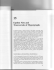### FÂ¡r.t` E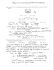### List of available CDs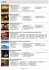### south east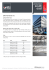### + m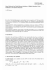### BLUE SEDUCTION L. 700 AZARO CHROME L. 850